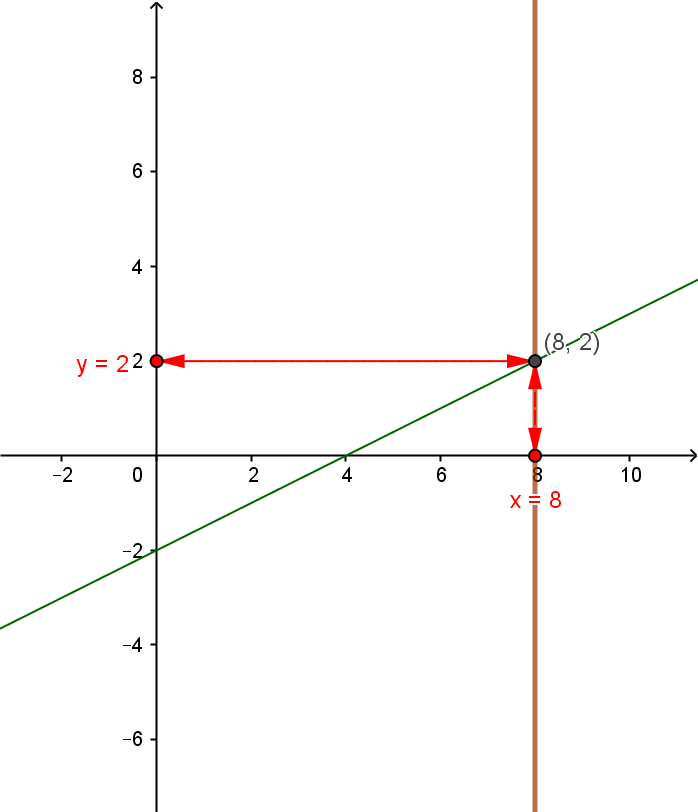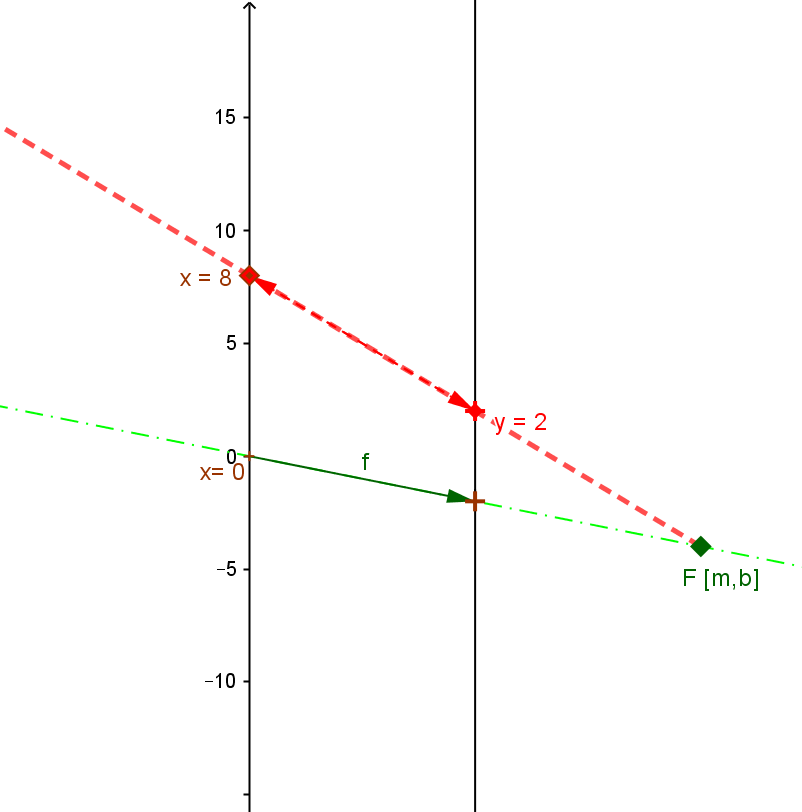Example LF.LEQ.4. Suppose $5x - 10y = 20$ (i) and $x=8$ (ii). Find $y$.
Solution A. Replace $x$ in the equation (i) by $8$ to give the equation $5*8 -10 y = 40- 10 y = 20$ (iii).  This leads to the equation $10 y = 20$ (iv) and thus the solution is $y = 2$.
Solution B. Express $y$ as a function of $x$ giving $y = f(x) = \frac 5 {10} x - \frac {20} {10} =\frac 1 2 x-2$ (v). Now evaluate $f(8) = \frac 1 2 8 - 2 =2$.

In Solution B we find the linear function being used to solve the problem merely by evaluation of the function. This evaluation can be visualized both on the graph and the mapping diagram.
 For the graph of $f$: Find $x=8$ on the X axis, then find the point on the graph of $f$ directly above (below) that point, $(8,2)$, determine it's second coordinate, $2$, and that is the desired value for $y$. For the mapping diagram of $f$: Find $x=8$ on the X axis, then find the focus point of $f$, $F= [\frac 1 2, -2]$ on the mapping diagram. Draw the line through F and the point $x=8$, to find the point of intersection of this line with the Y axis, $y=2$, which is the desired value for $y$.